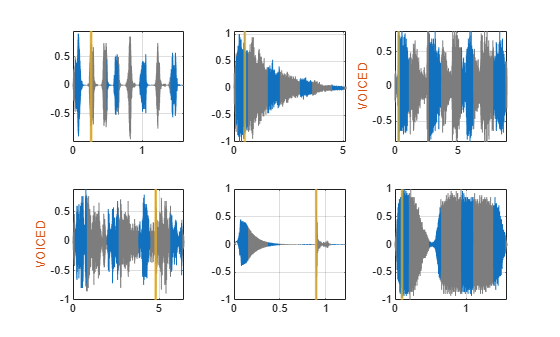# createDatastores

Create datastores pointing to signal and label data

## Syntax

``[sigdata,lbldata] = createDatastores(lss,lblnames)``

## Description

example

````[sigdata,lbldata] = createDatastores(lss,lblnames)` creates a datastore, `sigdata`, containing signal member data, and a datastore, `lbldata`, containing label data from labels specified in the string array `lblnames`. `createDatastores` does not apply to sublabels. Set `lblnames` to one or more parent label names to get the parent labels and the corresponding sublabel values.```

## Examples

collapse all

Load a labeled signal set containing recordings of whale songs.

```load whales lss```
```lss = labeledSignalSet with properties: Source: {2x1 cell} NumMembers: 2 TimeInformation: "sampleRate" SampleRate: 4000 Labels: [2x3 table] Description: "Characterize wave song regions" Use labelDefinitionsHierarchy to see a list of labels and sublabels. Use setLabelValue to add data to the set. ```

Display the labels for the first member of the set.

`lss.Labels(1,:)`
```ans=1×3 table WhaleType MoanRegions TrillRegions _________ ___________ ____________ Member{1} blue {3x2 table} {1x3 table} ```

Get the names of the labels in the set. Create a signal datastore with the signal information and an array datastore with the label information.

```lbls = getLabelNames(lss); [sgd,lbd] = createDatastores(lss,lbls)```
```sgd = signalDatastore with properties: MemberNames:{ 'Member{1}'; 'Member{2}' } Members: {2x1 cell} ReadSize: 1 SampleRate: 4000 ```
```lbd = ArrayDatastore with properties: ReadSize: 1 IterationDimension: 1 OutputType: "cell" ```

Display the labels for the first member of the set.

```lbls = read(lbd); lbls{:}```
```ans=1×3 table WhaleType MoanRegions TrillRegions _________ ___________ ____________ blue {3x2 table} {1x3 table} ```

Specify the path to a set of audio signals included as MAT-files with MATLAB®. Each file contains a signal variable and a sample rate. List the names of the files.

```folder = fullfile(matlabroot,"toolbox","matlab","audiovideo"); lst = dir(append(folder,"/*.mat")); nms = {lst(:).name}'```
```nms = 7x1 cell {'chirp.mat' } {'gong.mat' } {'handel.mat' } {'laughter.mat'} {'mtlb.mat' } {'splat.mat' } {'train.mat' } ```

Create a signal datastore that points to the specified folder. Set the sample rate variable name to `Fs`, which is common to all files. Generate a subset of the datastore that excludes the file `mtlb.mat`. Use the subset datastore as the source for a `labeledSignalSet` object.

```sds = signalDatastore(folder,"SampleRateVariableName","Fs"); sds = subset(sds,~strcmp(nms,"mtlb.mat")); lss = labeledSignalSet(sds);```

Create three label definitions to label the signals:

• Define a logical attribute label that is true for signals that contain human voices.

• Define a numeric point label that marks the location and amplitude of the maximum of each signal.

• Define a categorical region-of-interest (ROI) label to pick out nonoverlapping, uniform-length random regions of each signal.

Add the signal label definitions to the labeled signal set.

```vc = signalLabelDefinition("Voice",'LabelType','attribute', ... 'LabelDataType','logical','DefaultValue',false); mx = signalLabelDefinition("Maximum",'LabelType','point', ... 'LabelDataType','numeric'); rs = signalLabelDefinition("RanROI",'LabelType','ROI', ... 'LabelDataType','categorical','Categories',["ROI" "other"]); addLabelDefinitions(lss,[vc mx rs])```

Label the signals:

• Label `'handel.mat'` and `'laughter.mat'` as having human voices.

• Use the `islocalmax` function to find the maximum of each signal. Label its location and value.

• Use the `randROI` function to generate as many regions of length N/10 samples as can fit in a signal of length N given a minimum separation of N/6 samples between regions. Label their locations and assign them to the `ROI` category.

When labeling points and regions, convert sample values to time values. Subtract 1 to account for MATLAB® array indexing and divide by the sample rate.

```kj = 1; while hasdata(sds) [sig,info] = read(sds); fs = info.SampleRate; [~,fn] = fileparts(info.FileName); if fn=="handel" || fn=="laughter" setLabelValue(lss,kj,"Voice",true) end xm = find(islocalmax(sig,'MaxNumExtrema',1)); setLabelValue(lss,kj,"Maximum",(xm-1)/fs,sig(xm)) N = length(sig); rois = randROI(N,round(N/10),round(N/6)); setLabelValue(lss,kj,"RanROI",(rois-1)/fs,repelem("ROI",size(rois,1))) kj = kj+1; end```

Verify that only two signals contain voices.

`countLabelValues(lss,"Voice")`
```ans=2×3 table Voice Count Percent _____ _____ _______ false 4 66.667 true 2 33.333 ```

Verify that two signals have a maximum amplitude of 1.

`countLabelValues(lss,"Maximum")`
```ans=5×4 table Maximum Count Percent MemberCount ______________________ _____ _______ ___________ 0.80000000000000004441 1 16.667 1 0.89113331915798421612 1 16.667 1 0.94730769230769229505 1 16.667 1 1 2 33.333 2 1.0575668990330560071 1 16.667 1 ```

Verify that each signal has four nonoverlapping random regions of interest.

`countLabelValues(lss,"RanROI")`
```ans=2×4 table RanROI Count Percent MemberCount ______ _____ _______ ___________ ROI 24 100 6 other 0 0 0 ```

Create two datastores with the data in the labeled signal set:

`[sd,ld] = createDatastores(lss,["Voice" "RanROI" "Maximum"]);`

Use the information in the datastores to plot the signals and display their labels.

• Use a `signalMask` object to highlight the regions of interest in blue.

• Plot yellow lines to mark the locations of the maxima.

• Add a red axis label to the signals that contain human voices.

```tiledlayout flow while hasdata(sd) [sg,nf] = read(sd); lbls = read(ld); nexttile msk = signalMask(lbls{:}.RanROI{:},'SampleRate',nf.SampleRate); plotsigroi(msk,sg) colorbar off xlabel('') xline(lbls{:}.Maximum{:}.Location, ... 'LineWidth',2,'Color','#EDB120') if lbls{:}.Voice{:} ylabel('VOICED','Color','#D95319') end end``````function roilims = randROI(N,wid,sep) num = floor((N+sep)/(wid+sep)); hq = histcounts(randi(num+1,1,N-num*wid-(num-1)*sep),(1:num+2)-1/2); roilims = (1 + (0:num-1)*(wid+sep) + cumsum(hq(1:num)))' + [0 wid-1]; end```

## Input Arguments

collapse all

Labeled signal set, specified as a `labeledSignalSet` object.

Example: ```labeledSignalSet({randn(100,1) randn(10,1)},signalLabelDefinition('female'))``` specifies a two-member set of random signals containing the attribute `'female'`.

Label names, specified as a character vector, a string scalar, a cell array of character vectors, or a string array.

Data Types: `char` | `string`

## Output Arguments

collapse all

Signal data, returned as a `signalDatastore` object or an `audioDatastore` (Audio Toolbox) object.

Label data, returned as an `arrayDatastore` object.

## Version History

Introduced in R2021a